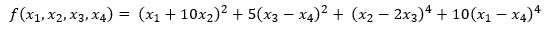# SQL Server NLMIN function

NLMIN
Updated: 14 July 2020

Use the table-valued function NLMIN to find the minimum of a function using the Nelder-Mead method.

Syntax
SELECT * FROM [wct].[NLMIN] (
<@Func, nvarchar(max),>
,<@VarNames, nvarchar(max),>
,<@StartVals, nvarchar(max),>
,<@ReqMin, float,>
,<@StepVals, nvarchar(max),>
,<@konvge, int,>
,<@kcount, int,>)
Arguments
 Input Name Description @Func The function to be evaluated, as a string. The function must be in the form of a SELECT statement. @VarNames The names of the variables @StartVals The starting point for the minimization @ReqMin The required minimum value @StepVals Step size for each variable @konvge The convergence check is carried out every KONVGE iterations @kcount The maximum number of function evaluations
Return Type
TABLE (
[VarName] [nvarchar](max) NULL,
[val] [float] NULL
)
Remarks
• The returned table includes the variable names and values which are estimated to minimize the function.
• If @Func is not a valid SELECT statement then NULL is returned.
• The returned table includes the variable YNEWLO which was the minimum value calculated.
• The retuned table includes ICOUNT, the number of function evaluations.
• The returned table include NUMRES, the number of restarts.
• The retuned table include IFAULT, and error indicator
• 0 — no errors detected
• 1 — @ReqMin, @StartVals or @konvge has an illegal value
• 2 — iteration was terminated because @kcount was exceeded without convergence.
Examples
Example #1

Calculate the minimum ofOur initial estimate is (3,-1,0,1).

DECLARE @func as varchar(max) = N'
SELECT
POWER(@x1+10*@x2,2)
+5*POWER(@x3-@x4,2)
+POWER(@x2 - 2*@x3,4)
+10*POWER(@x1 - @x4, 4)';
DECLARE @varnames as varchar(max) = N'@x1,@x2,@x3,@x4';
DECLARE @startvals as varchar(max) = N'3.0,-1.0,0.0,1.0';
DECLARE @reqmin as float = 1e-08;
DECLARE @stepvals as varchar(max) = N'1.0,1.0,1.0,1.0';
DECLARE @konvge as int = 10;
DECLARE @kcount as int = 500;

SELECT
VarName,
val
FROM
wct.NLMIN(
@func,
@varnames,
@startvals,
@reqmin,
@stepvals,
@konvge,
@kcount
);

This produces the following result.Copyright 2008-2022 Westclintech LLC         Privacy Policy        Terms of Service